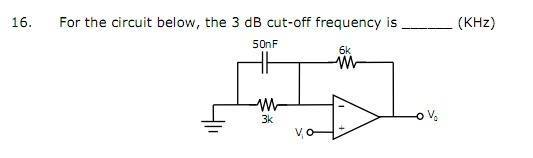# Need help in opamp oscillator

• naman chauhan

#### naman chauhan

Hi everyone,I was trying to solve this problem. Here at calculate 3 db frequency the gain should me 1/sqrt(2) times of the maximum voltage gain.
So I calculated maximum gain which is 1+6/3=3 ( capacitor will be open for maximum gain). At 3db gain will be 3/1.414
3/1.414=(1+6k/(3k||(1/jwc))). Then I solved for the frequency. But I am getting the wrong answer. Can somebody please tell me that wether I am making a mathematical mistake or applying wrong concept.

What happens at high frequencies when the capacitor is a short.

At heigh frequency the inverting terminal is connected to ground. And since it is a negative feed back circuit so the output will also be 0.

Nope. The op amp wants the voltage between + and - to be zero.

Yes it does. But at heigh frequency the capacitor is short and 100% voltage is fended back to the opamp. That's why output will be zero

Yes it does. But at heigh frequency the capacitor is short and [strike]100%[/strike] 0% voltage is fended back to the opamp. That's why output will be ...
Fixed.Hi everyone,
View attachment 61654

Have you figured out what kind of circuit this is? What is meant by "cutoff frequency" for this circuit? The term 'cutoff frequency' is a very poor one, since the gain is finite (=3) at 0 Hz and approaches infinity for very high frequencies.

So the 'cutoff frequency' is 3db above the dc gain of 3.
That frequency is not formed by the 3K resistor only.

I think that the gain is o at very heigh frequency and as gain vary from 3 to 0 the cutoff frequency will be where the gain will be 3/1.414

I think that the gain is o at very heigh frequency and as gain vary from 3 to 0 the cutoff frequency will be where the gain will be 3/1.414

I think that the gain is very high at very high frequency.

At very heigh frequency the inverting terminal is connected to ground through short capacitor and now if we apply vi then there is a potential difference between the input terminals of opamp so it will produce heigh output. But here at the same time we are getting feedback through 6k resistor Wouldn't that effect the gain of the opamp.

Write the equation for the gain of the circuit and you will see.

If you remember the gain for non-inverting input = 1 + Zf/Zi it is obvious that at high frequencies Zi → 0.

please explain how one gets feedback through the 6K resistor if the - pin side is connected to ground? What voltage can the opamp output that will raise ground to 1V?

Ok I get that gain become very heigh at heigh frequency. Sorry I misunderstood the concept. But if gain is varying between 3 to very heigh value then what's the meaning of 3db frequency?

I'm going to let you chew on that one. Reread the responses now that you understand the basics.

Ok I get that gain become very heigh at heigh frequency. Sorry I misunderstood the concept. But if gain is varying between 3 to very heigh value then what's the meaning of 3db frequency?